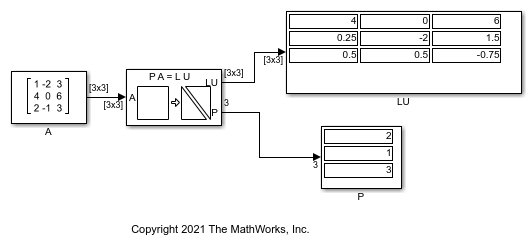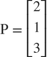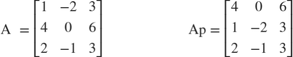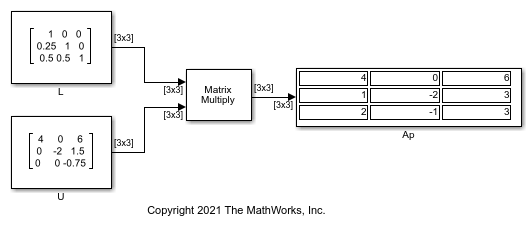# Factorize Matrix Using LU Factorization Block

Factorize a square matrix into upper and lower submatrices using the LU Factorization block.

The LU Factorization block factors the matrix Ap into upper and lower triangular submatrices U and L, where Ap is the row-permuted version of input matrix A.P is the permutation index vector which determines how the block reorganizes the input matrix A to form the permuted matrix Ap. With, the block interchanges the first and second rows of the input matrix A to form the permuted matrix Ap.The LU output is a composite matrix containing the two submatrix factors L and U.The product of L and U matrices equals Ap. You can verify this using the following model.